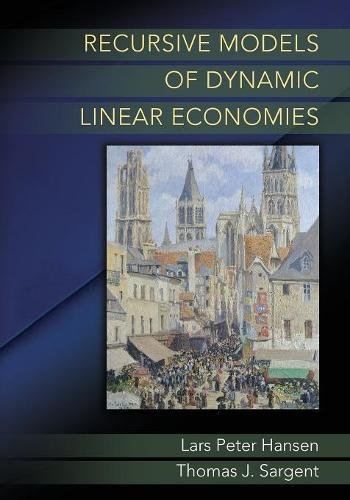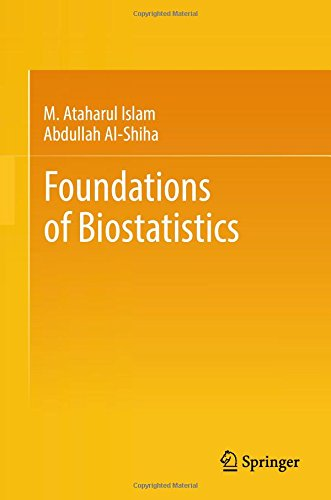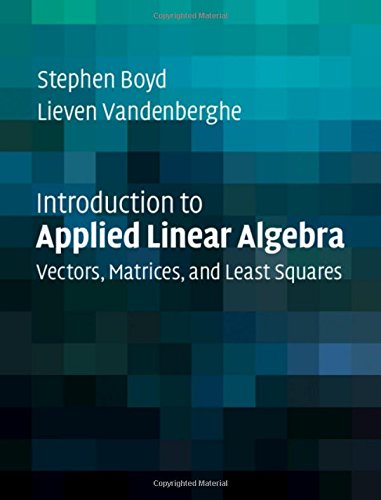# Recursive Models of Dynamic Linear Economies (The Gorman Lectures in Economics)A common set of mathematical tools underlies dynamic optimization, dynamic estimation, and filtering. In Recursive Models of Dynamic Linear Economies, Lars Peter Hansen and Thomas Sargent use these tools to create a class of econometrically tractable models of prices and quantities. They present examples from microeconomics, macroeconomics, and asset pricing. The models are cast in terms of a representative consumer. While Hansen and Sargent demonstrate the analytical benefits acquired when an analysis with a representative consumer is possible, they also characterize the restrictiveness of assumptions under which a representative household justifies a purely aggregative analysis. Hansen and Sargent unite economic theory with a workable econometrics while going beyond and beneath demand and supply curves for dynamic economies. They construct and apply competitive equilibria for a class of linear-quadratic-Gaussian dynamic economies with complete markets. Their book, based on the 2012 Gorman lectures, stresses heterogeneity, aggregation, and how a common structure unites what superficially appear to be diverse applications. An appendix describes MATLAB programs that apply to the book’s calculations.
Buy the book Recursive Models of Dynamic Linear Economies (The Gorman Lectures in Economics) from Ideakart.com.

# Foundations of BiostatisticsThis book offers a comprehensive guide to essential techniques and methods in biostatistics, addressing the underlying concepts to aid in comprehension. The use of biostatistics techniques has increased manifold in the recent past, due to their suitability for applications in a wide range of problems in various fields. This book helps learners grasp the materials in detail, equipping them to use biostatistics techniques independently and confidently. The book starts with a summary of background materials, followed by methods and techniques. As such, with only minimum guidance from teachers, this book can provide materials for self-learning of biostatistics techniques with a deeper level of understanding.  The first two chapters focus on fundamental concepts, sources of data, data types, organization of data, and descriptive statistics, followed by the basic probability concepts, distributions and sampling distributions needed in order to combine descriptive statistics with inferential techniques. Estimation and tests of hypotheses are illustrated in two separate chapters. Important measures of association, linear regression, analysis of variance and logistic regression, and proportional hazards models are then presented systematically, ensuring that the book covers the topics most essential to students and users of biostatistics in connection with a wide range of applications in various fields. The book has been carefully structured, and the content is presented in a sequence covering the essential background in a highly systematic manner, supporting the learning process by presenting theory and applications that complement one another.

# Introduction to Applied Linear Algebra: Vectors, Matrices, and Least SquaresThis groundbreaking textbook combines straightforward explanations with a wealth of practical examples to offer an innovative approach to teaching linear algebra. Requiring no prior knowledge of the subject, it covers the aspects of linear algebra – vectors, matrices, and least squares – that are needed for engineering applications, discussing examples across data science, machine learning and artificial intelligence, signal and image processing, tomography, navigation, control, and finance. The numerous practical exercises throughout allow students to test their understanding and translate their knowledge into solving real-world problems, with lecture slides, additional computational exercises in Julia and MATLAB, and data sets accompanying the book online. It is suitable for both one-semester and one-quarter courses, as well as self-study, this self-contained text provides beginning students with the foundation they need to progress to more advanced study.
Buy the book Introduction to Applied Linear Algebra: Vectors, Matrices, and Least Squares from Ideakart.com.